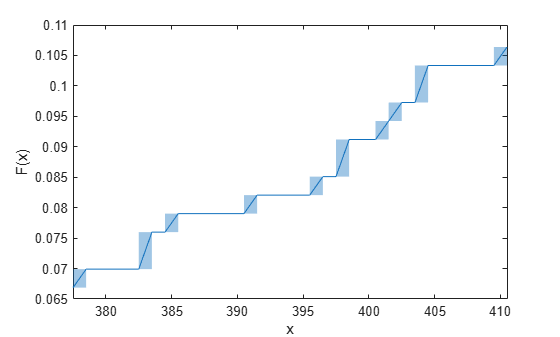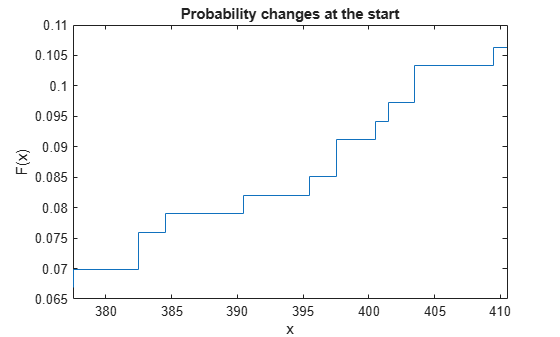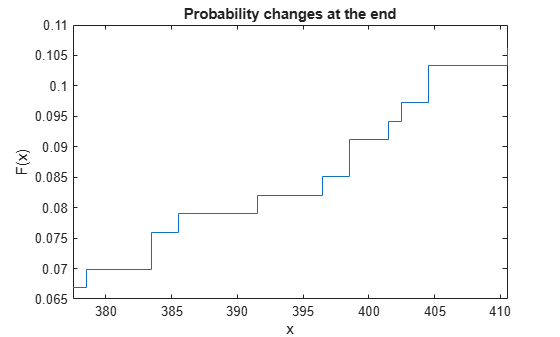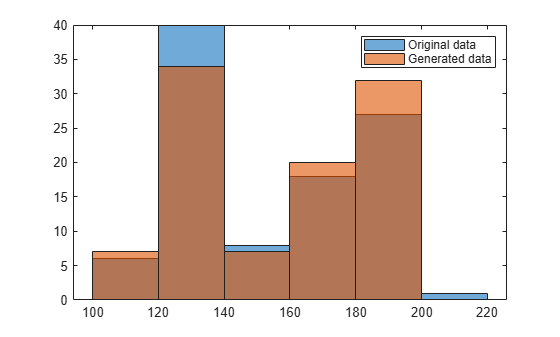# ecdf

Empirical cumulative distribution function

## Syntax

``````[f,x] = ecdf(y)``````
``````[f,x] = ecdf(y,Name,Value)``````
``````[f,x,flo,fup] = ecdf(___)``````
``ecdf(___)``
``ecdf(ax,___)``

## Description

example

``````[f,x] = ecdf(y)``` returns the empirical cumulative distribution function `f`, evaluated at `x`, using the data in `y`.```

example

``````[f,x] = ecdf(y,Name,Value)``` specifies additional options using one or more name-value arguments. For example, `'Function','survivor'` specifies the type of function for `f` as a survivor function.```

example

``````[f,x,flo,fup] = ecdf(___)``` also returns the lower and upper confidence bounds for the evaluated function values, using any of the input argument combinations in the previous syntaxes. This syntax is not valid for interval-censored data.```

example

````ecdf(___)` produces a stairstep graph of the evaluated function. The function visualizes interval estimates for interval-censored data using shaded rectangles. You can specify `'Bounds','on'` to include the confidence bounds in the graph for fully observed, left-censored, right-censored, and double-censored data.```
````ecdf(ax,___)` plots on the axes specified by `ax` instead of the current axes (`gca`).```

## Examples

collapse all

Compute the Kaplan-Meier estimate of the empirical cumulative distribution function (cdf) for simulated survival data.

Generate survival data from a Weibull distribution with parameters 3 and 1.

```rng('default') % For reproducibility failuretime = random('wbl',3,1,15,1);```

Compute the Kaplan-Meier estimate of the empirical cdf for survival data.

```[f,x] = ecdf(failuretime); [f,x]```
```ans = 16×2 0 0.0895 0.0667 0.0895 0.1333 0.1072 0.2000 0.1303 0.2667 0.1313 0.3333 0.2718 0.4000 0.2968 0.4667 0.6147 0.5333 0.6684 0.6000 1.3749 ⋮ ```

Plot the estimated empirical cdf.

`ecdf(failuretime)`Generate right-censored survival data and compare the empirical cumulative distribution function (cdf) with the known cdf.

Generate failure times from an exponential distribution with a mean failure time of 15.

```rng('default') % For reproducibility y = exprnd(15,75,1);```

Generate drop-out times from an exponential distribution with a mean failure time of 30.

`d = exprnd(30,75,1);`

Generate the observed failure times, that is, the minimum of the generated failure times and the drop-out times.

`t = min(y,d);`

Create a logical array containing generated failure times that are larger than the drop-out times. The data for which this condition is true is censored.

`censored = (y>d);`

Compute the empirical cdf and confidence bounds.

`[f,x,flo,fup] = ecdf(t,'Censoring',censored);`

Plot the empirical cdf and confidence bounds.

```ecdf(t,'Censoring',censored,'Bounds','on') hold on```Superimpose a plot of the known population cdf.

```xx = 0:.1:max(t); yy = 1-exp(-xx/15); plot(xx,yy,'g-','LineWidth',2) axis([0 max(t) 0 1]) legend('Empirical cdf','Lower confidence bound', ... 'Upper confidence bound','Known population cdf', ... 'Location','southeast') hold off```Generate survival data and plot the empirical survivor function with 99% confidence bounds.

Generate lifetime data from a Weibull distribution with parameters 100 and 2.

```rng('default') % For reproducibility R = wblrnd(100,2,100,1);```

Plot the empirical survivor function for the data with 99% confidence bounds.

```ecdf(R,'Function','survivor','Alpha',0.01,'Bounds','on') hold on```Superimpose a plot of the Weibull survivor function.

```x = 1:1:250; wblsurv = 1-cdf('weibull',x,100,2); plot(x,wblsurv,'g-','LineWidth',2) legend('Empirical survivor function','Lower confidence bound', ... 'Upper confidence bound','Weibull survivor function', ... 'Location','northeast')```The Weibull survivor function based on the actual distribution is within the confidence bounds.

Compute and plot the cumulative hazard function of simulated double-censored survival data.

Generate failure times from a Birnbaum-Saunders distribution.

```rng('default') % For reproducibility failuretime = random('BirnbaumSaunders',0.3,1,[100,1]);```

Assume that the study starts at time 0.1 and the ends at time 0.9. The assumption implies that failure times less than 0.1 are left censored, and failure times greater than 0.9 are right censored.

Create a vector in which each element indicates the censorship status of the corresponding observation in `failuretime`. Use –1, 1, and 0 to indicate left-censored, right-censored, and fully observed observations, respectively.

```L = 0.1; U = 0.9; left_censored = (failuretime<L); right_censored = (failuretime>U); c = right_censored - left_censored;```

Plot the empirical cumulative hazard function for the data with 95% confidence bounds.

```ecdf(failuretime,'Function','cumulative hazard', ... 'Censoring',c,'Bounds','on')```Compute and plot the empirical cdf of interval-censored data.

Load the `cities` data set. The data includes ratings for nine different indicators of the quality of life in 329 US cities: climate, housing, health, crime, transportation, education, arts, recreation, and economics. For each indicator, a higher rating is better.

`load cities`

Select the first indicator (climate) as sample data.

`Y = ratings(:,1);`

Assume that the indicators in `Y` are the values rounded to the nearest integer. Then, you can treat values in `Y` as interval-censored observations. An observation `y` in `Y` indicates that the actual rating is between `y–0.5` and `y+0.5`.

Create a matrix in which each row represents the interval surrounding each integer in `Y`.

`intervalY = [Y-0.5, Y+0.5];`

Compute the empirical cdf values.

`[f,x] = ecdf(intervalY);`

Plot the empirical cdf values.

```figure ecdf(intervalY)```Zoom into a smaller region to see the interval estimates.

```idx_roi = 21:30; xlim([x(idx_roi(1),1) x(idx_roi(end),2)])```Display the corresponding `x` and `f` values.

```table(idx_roi',x(idx_roi,:),f(idx_roi,:), ... 'VariableNames',{'Index','x','Empirical cdf F(x)'})```
```ans=10×3 table Index x Empirical cdf F(x) _____ ______________ __________________ 21 377.5 378.5 0.069909 22 382.5 383.5 0.075988 23 384.5 385.5 0.079027 24 390.5 391.5 0.082067 25 395.5 396.5 0.085106 26 397.5 398.5 0.091185 27 400.5 401.5 0.094225 28 401.5 402.5 0.097264 29 403.5 404.5 0.10334 30 409.5 410.5 0.10638 ```

The shaded rectangles indicate the change of empirical cdf values F(x) within the corresponding intervals. For example, the second shaded rectangle from the left in the zoomed plot corresponds to the interval (382.5,383.5]. F(382.5) is 0.075988, F(383.5) is 0.079027, and the change from 0.075988 to 0.079027 occurs in the interval (382.5,383.5]. The exact timing of the change is uncertain.

You can plot the interval estimates in different ways. If you assume that the probability change occurs at the start of each interval, you can plot the F(x) values using the first column of x.

```figure stairs(x(:,1),f) title('Probability changes at the start') xlabel('x') ylabel('F(x)') xlim([x(idx_roi(1),1) x(idx_roi(end),2)])```Alternatively, you can plot the F(x) values using the second column of x with the assumption that the probability change occurs at the end of each interval.

```figure stairs(x(:,2),f) title('Probability changes at the end') xlabel('x') ylabel('F(x)') xlim([x(idx_roi(1),1) x(idx_roi(end),2)])```Combine the previous two plots to visualize the intervals.

```figure stairs(x(:,1),f) hold on stairs(x(:,2),f) title('Probability changes in the interval') xlabel('x') ylabel('F(x)') xlim([x(idx_roi(1),1) x(idx_roi(end),2)]) hold off```Compute the empirical cumulative distribution function (cdf) for data, and create a piecewise linear distribution object using an approximation to the empirical cdf.

Load the sample data. Visualize the patient weight data using a histogram.

```load patients histogram(Weight(strcmp(Gender,'Female'))) hold on histogram(Weight(strcmp(Gender,'Male'))) legend('Female','Male')```The histogram shows that the data has two modes, one for female patients and one for male patients.

Compute the empirical cdf for the data.

`[f,x] = ecdf(Weight);`

Construct a piecewise linear approximation to the empirical cdf by taking a value every five points.

```f = f(1:5:end); x = x(1:5:end);```

Plot the empirical cdf and the approximation.

```figure ecdf(Weight) hold on plot(x,f,'ko-','MarkerFace','r') legend('Empirical cdf','Piecewise linear approximation', ... 'Location','best')```Create a piecewise linear probability distribution object using the piecewise approximation of the empirical cdf.

`pd = makedist('PiecewiseLinear','x',x,'Fx',f)`
```pd = PiecewiseLinearDistribution F(111) = 0 F(118) = 0.05 F(124) = 0.13 F(130) = 0.25 F(135) = 0.37 F(142) = 0.5 F(163) = 0.55 F(171) = 0.61 F(178) = 0.7 F(183) = 0.82 F(189) = 0.94 F(202) = 1 ```

Generate 100 random numbers from the distribution.

```rng('default') % For reproducibility rw = random(pd,[100,1]);```

Plot the random numbers to visually compare their distribution to the original data.

```figure histogram(Weight) hold on histogram(rw) legend('Original data','Generated data')```The random numbers generated from the piecewise linear distribution have the same bimodal distribution as the original data.

## Input Arguments

collapse all

Sample data and censorship information, specified as a vector of sample data or a two-column matrix of sample data and censorship information.

You can specify the censorship information for the sample data by using either the `y` argument or the `Censoring` name-value argument. `ecdf` ignores the `Censoring` argument value if `y` is a two-column matrix.

Specify `y` as a vector or a two-column matrix depending on the censorship types of the observations in `y`.

• Fully observed data — Specify `y` as a vector of sample data.

• Data that contains fully observed, left-censored, or right-censored observations — Specify `y` as a vector of sample data, and specify the `Censoring` name-value argument as a vector that contains the censorship information for each observation. The `Censoring` vector can contain 0, –1, and 1, which refer to fully observed, left-censored, and right-censored observations, respectively.

• Data that includes interval-censored observations — Specify `y` as a two-column matrix of sample data and censorship information. Each row of `y` specifies the range of possible survival or failure times for each observation, and can have one of these values.

• `[t,t]` — Fully observed at `t`

• `[–Inf,t]` — Left-censored at `t`

• `[t,Inf]` — Right-censored at `t`

• `[t1,t2]` — Interval-censored between `[t1,t2]`, where `t1` < `t2`

`ecdf` ignores `NaN` values in `y`. Additionally, any `NaN` values in the censoring vector (`Censoring`) or frequency vector (`Frequency`) cause `ecdf` to ignore the corresponding rows in `y`.

Data Types: `single` | `double`

Target axes for the figure to which `ecdf` plots, specified as an `Axes` object.

For instance, if `h` is a target `Axes` object for a figure, then `ecdf` can plot to that figure as shown in the following example.

Example: `ecdf(h,x)`

### Name-Value Arguments

Specify optional pairs of arguments as `Name1=Value1,...,NameN=ValueN`, where `Name` is the argument name and `Value` is the corresponding value. Name-value arguments must appear after other arguments, but the order of the pairs does not matter.

Before R2021a, use commas to separate each name and value, and enclose `Name` in quotes.

Example: ```'Censoring',c,'Function','cumulative hazard','Alpha',0.025,'Bounds','on'``` instructs `ecdf` to return the cumulative hazard function and the 97.5% confidence bounds, accounting for the censored data specified by vector `c`.

Type of function returned by `ecdf`, specified as one of these values.

ValueDescription
`'cdf'` (default)Cumulative distribution function (cdf)
`'survivor'`Survivor Function
`'cumulative hazard'`Cumulative Hazard Function

Example: `'Function','cumulative hazard'`

Indicator of censored data, specified as a vector consisting of 0, –1, and 1, which indicate fully observed, left-censored, and right-censored observations, respectively. Each element of the `Censoring` value indicates the censorship status of the corresponding observation in `y`. The `Censoring` value must have the same size as `y`. The default is a vector of 0s, indicating all observations are fully observed.

You cannot specify interval-censored observations using this argument. If the sample data includes interval-censored observations, specify `y` using a two-column matrix. `ecdf` ignores the `Censoring` value if `y` is a two-column matrix.

`ecdf` ignores any `NaN` values in the censoring vector. Additionally, any `NaN` values in `y` or the frequency vector (`Frequency`) cause `ecdf` to ignore the corresponding values in the censoring vector.

Example: `'Censoring',censored`, where `censored` is a vector that contains censorship information.

Data Types: `logical` | `single` | `double`

Frequency of observations, specified as a vector of nonnegative integer counts that has the same number of rows as `y`. The `j`th element of the `Frequency` value gives the number of times the `j`th row of `y` was observed. The default is a vector of 1s, indicating one observation per row of `y`.

`ecdf` ignores any `NaN` values in this frequency vector. Additionally, any `NaN` values in `y` or the censoring vector (`Censoring`) cause `ecdf` to ignore the corresponding values in the frequency vector.

Example: `'Frequency',freq`, where `freq` is a vector that contains the observation frequencies.

Data Types: `single` | `double`

Maximum number of iterations, specified as a positive integer. This argument is valid only for double-censored data and interval-censored data.

Example: `'IterationLimit',1e5`

Data Types: `single` | `double`

Termination tolerance on the function value `f`, specified as a positive scalar. This argument is valid only for double-censored data and interval-censored data.

Example: `'Tolerance',1e-5`

Data Types: `single` | `double`

Frequency of the iterative convex minorant (ICM) step, specified as a positive integer. This argument is valid only for interval-censored data.

`ecdf` uses the expectation-maximization iterative convex minorant (EMICM) algorithm  to compute the output `f` for interval-censored data. The EMICM algorithm uses either the EM algorithm or the ICM algorithm at each iteration. `ecdf` runs the ICM step every specified number of iterations. For example, by default, `ecdf` iterates the EM step nine times, runs the ICM step once, and then goes back to the EM step.

Example: `'ICMFrequency',1`

Data Types: `single` | `double`

Significance level for the confidence interval of the evaluated function, specified as a scalar in the range (0,1). The default is 0.05 for 95% confidence. For a given value `alpha`, the confidence level is `100(1 – Alpha)`%.

This argument is not valid for interval-censored data.

Example: `'Alpha',0.01` specifies the confidence level as 99%.

Data Types: `single` | `double`

Indicator for including the confidence bounds in the plot, specified as one of these values.

ValueDescription
`'off'` (default)Omit the confidence bounds.
`'on'` Include the confidence bounds.

This argument is not valid for interval-censored data.

Note

This argument is valid only for plotting.

Example: `'Bounds','on'`

## Output Arguments

collapse all

Function values evaluated at the points or intervals in `x`, returned as a column vector.

• The point estimate indicates that the function value at `x(i)` is `f(i)`.

• The interval estimate indicates that the function value changes from `f(i–1)` to `f(i)` within the interval (`x(i,1)`,`x(i,2)`]. The exact timing of the change is uncertain. For an example, see Empirical cdf of Interval-Censored Data.

The function type of `f` can be the cdf (default), Survivor Function, or Cumulative Hazard Function, as specified by the `Function` name-value argument.

Evaluation points or intervals, specified as a column vector or a two-column matrix, respectively.

• `ecdf` returns a column vector for fully observed, left-censored, right-censored, and double-censored data.

• For fully observed, left-censored, and right-censored data, `ecdf` removes values for censored observations from `y`, sorts the remaining values, removes duplicate values in the sorted values, and saves the results to the output `x`.

• For double-censored data, `ecdf` determines which values of `y` correspond to the event times, sorts the values, removes duplicate values in the sorted values, and saves the results to the output `x`.

The output `x` includes the minimum value of `y` as its first two values. These two values are useful for plotting the outputs of `ecdf` using the `stairs` function.

• `ecdf` returns a two-column matrix for interval-censored data. `ecdf` evaluates the function values `f` at intervals called Turnbull intervals. For details, see Algorithms.

Lower confidence bound for the evaluated function, returned as a column vector. `ecdf` computes the bound for each observation. `flo` is not a simultaneous bound for the curve.

This argument is not valid for interval-censored data.

Upper confidence bound for the evaluated function, returned as a column vector. `ecdf` computes the bound for each observation. `fup` is not a simultaneous bound for the curve.

This argument is not valid for interval-censored data.

collapse all

### Censorship Types

`ecdf` supports left-censored, right-censored, and interval-censored observations.

• Left-censored observation at time `t` — The event occurred before time `t`, and the exact event time is unknown.

• Right-censored observation at time `t` — The event occurred after time `t`, and the exact event time is unknown.

• Interval-censored observation within the interval `[t1,t2]` — The event occurred after time `t1` and before time `t2`, and the exact event time is unknown.

Double-censored data includes both left-censored and right-censored observations.

### Survivor Function

The survival function is the probability of survival as a function of time. It is also called the survivor function.

The survival function gives the probability that the survival time of an individual exceeds a certain value. Because the cumulative distribution function F(t) is the probability that the survival time is less than or equal to a given point t in time, the survival function for a continuous distribution S(t) is the complement of the cumulative distribution function: S(t) = 1 – F(t).

### Cumulative Hazard Function

The hazard function h(t) is the instantaneous failure rate of an individual conditioned on the fact that the individual survived until a given time. The cumulative hazard function H(t) is the cumulative hazard up to time t.

`$h\left(t\right)=\underset{\Delta t\to 0}{\mathrm{lim}}\frac{P\left(t\le T`

`$H\left(t\right)={\int }_{0}^{t}h\left(u\right)du.$`

The hazard function always takes a positive value. However, these values do not correspond to probabilities and can be greater than 1.

You can obtain the cumulative hazard function values from the Survivor Function S(t) using the relation S(t) = exp(–H(t)).

## Algorithms

`ecdf` computes the function values (`f`) and the confidence bounds (`flo` and `fup`) using different algorithms, depending on the censorship information. The function type of `f` can be the cdf (default), Survivor Function, or Cumulative Hazard Function, as specified by the `Function` name-value argument.

Censorship TypeAlgorithm for `f`Algorithm for `flo` and `fup`
Right-censored data, which contains fully observed or right-censored observations
• Use the Kaplan-Meier estimator for the cdf and survivor function values.

The Kaplan-Meier estimator $\stackrel{^}{S}\left(t\right)$ is given by

`$\stackrel{^}{S}\left(t\right)=\prod _{{t}_{i}`

where ri is the number of observations at risk at time ti, and di is the number of failures at time ti. For more details, see Kaplan-Meier Method.

• Use the Nelson-Aalen estimator for the cumulative hazard function values.

The Nelson-Aalen estimator is given by

`$\stackrel{^}{H}\left(t\right)=\sum _{{t}_{i}`

Use Greenwood’s formula, which is an approximation for the variance of the Kaplan-Meier estimator.

The variance estimate is given by

`$V\left(\stackrel{^}{S}\left(t\right)\right)={\stackrel{^}{S}}^{2}\left(t\right)\sum _{{t}_{i}`

Left-censored data, which contains fully observed or left-censored observations

Use the Kaplan-Meier estimator.

Use Greenwood's formula.

Double-censored data, which includes both right-censored and left-censored observations

Use Turnbull's algorithm . You can specify the maximum number of iterations (`IterationLimit`) and the termination tolerance on the function value (`Tolerance`) for the algorithm.

Use the Fisher information matrix.

Interval-censored data, which includes interval-censored observations
• Use the expectation-maximization iterative convex minorant (EMICM) algorithm . The EMICM algorithm uses either the EM algorithm or the ICM algorithm at each iteration. The `ICMFrequency` name-value argument determines the frequency of the ICM algorithm. `ecdf` runs the ICM step every specified number of iterations. By default, `ecdf` iterates the EM step nine times, runs the ICM step once, and then goes back to the EM step. You can specify the maximum number of iterations (`IterationLimit`) and the termination tolerance on the function value (`Tolerance`) for the algorithm.

• `ecdf` constructs mutually disjoint intervals, called Turnbull intervals, from the two-column matrix data `y`, and returns the Turnbull intervals (`x`) and the estimates (`f`) at the intervals. The left bounds of the intervals are from the first column of `y`, and the right bounds of the intervals are from the second column of `y`. For a fully observed observation (for the row with two of the same values `[t t]`), the function converts `[t t]` to `[t–eps(t) t]` to create an interval with nonzero length before constructing the Turnbull intervals.

Not supported

 Cox, D. R., and D. Oakes. Analysis of Survival Data. London: Chapman & Hall, 1984.

 Lawless, J. F. Statistical Models and Methods for Lifetime Data. 2nd ed., Hoboken, NJ: John Wiley & Sons, Inc., 2003.

 Klein, John P., and Melvin L. Moeschberger. Survival Analysis: Techniques for Censored and Truncated Data. 2nd ed. Statistics for Biology and Health. New York: Springer, 2003.

 Turnbull, Bruce W. "Nonparametric Estimation of a Survivorship Function with Doubly Censored Data." Journal of the American Statistical Association 69, No. 345 (1974): 169–73.

 Anderson-Bergman, Clifford. "An Efficient Implementation of the EMICM Algorithm for the Interval Censored NPMLE." Journal of Computational and Graphical Statistics 26, no. 2 (April 3, 2017): 463–67.

 Ware, James H., and David L. Demets. "Reanalysis of Some Baboon Descent Data." Biometrics 32, no. 2 (June 1976): 459–63.